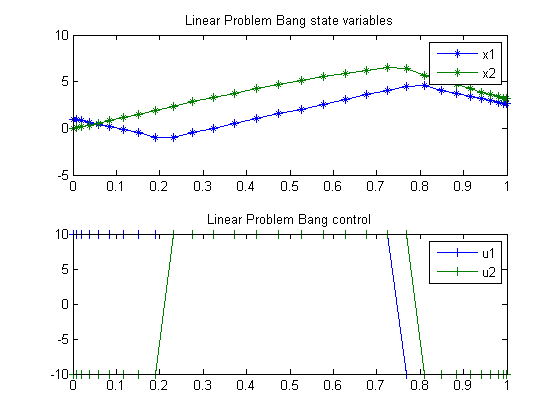60  Linear Problem with Bang Bang Control

Problem 5a: Miser3 manual

60.1  Problem description

Find u over t in [0; 1 ] to minimize

J =
 1 0
−6*x1−12*x2+3*u1+u2 dt

subject to:

 dx1 dt
= u2
 dx2 dt
= −x1+u1
 x1(0) = 1
 x2(0) = 0
 |u| <= 10

Reference: 

60.2  Problem setup

toms t
p = tomPhase('p', t, 0, 1, 30);
setPhase(p);

tomStates x1 x2
tomControls u1 u2

% Initial guess
x0 = {icollocate({x1 == 1; x2 == 0})
collocate({u1 == 0; u2 == 0})};

% Box constraints
cbox = {-10 <= icollocate(x1) <= 10
-10 <= icollocate(x2) <= 10
-10 <= collocate(u1)  <= 10
-10 <= collocate(u2)  <= 10};

% Boundary constraints
cbnd = initial({x1 == 1; x2 == 0});

% ODEs and path constraints
ceq = collocate({dot(x1) == u2
dot(x2) == -x1+u1});

% Objective
objective = integrate(-6*x1-12*x2+3*u1+u2);

60.3  Solve the problem

options = struct;
options.name = 'Linear Problem Bang';
solution = ezsolve(objective, {cbox, cbnd, ceq}, x0, options);
t  = subs(collocate(t),solution);
x1 = subs(collocate(x1),solution);
x2 = subs(collocate(x2),solution);
u1 = subs(collocate(u1),solution);
u2 = subs(collocate(u2),solution);
Problem type appears to be: lp
Starting numeric solver
===== * * * =================================================================== * * *
TOMLAB - Tomlab Optimization Inc. Development license  999001. Valid to 2011-02-05
=====================================================================================
Problem: ---  1: Linear Problem Bang            f_k     -41.377652213983296000
sum(|constr|)      0.000000000005182483
f(x_k) + sum(|constr|)    -41.377652213978116000
f(x_0)      0.000000000000000000

Solver: CPLEX.  EXIT=0.  INFORM=1.
CPLEX Dual Simplex LP solver
Optimal solution found

FuncEv   63 Iter   63
CPU time: 0.015625 sec. Elapsed time: 0.016000 sec.

60.4  Plot result

subplot(2,1,1)
plot(t,x1,'*-',t,x2,'*-');
legend('x1','x2');
title('Linear Problem Bang state variables');

subplot(2,1,2)
plot(t,u1,'+-',t,u2,'+-');
legend('u1','u2');
title('Linear Problem Bang control');